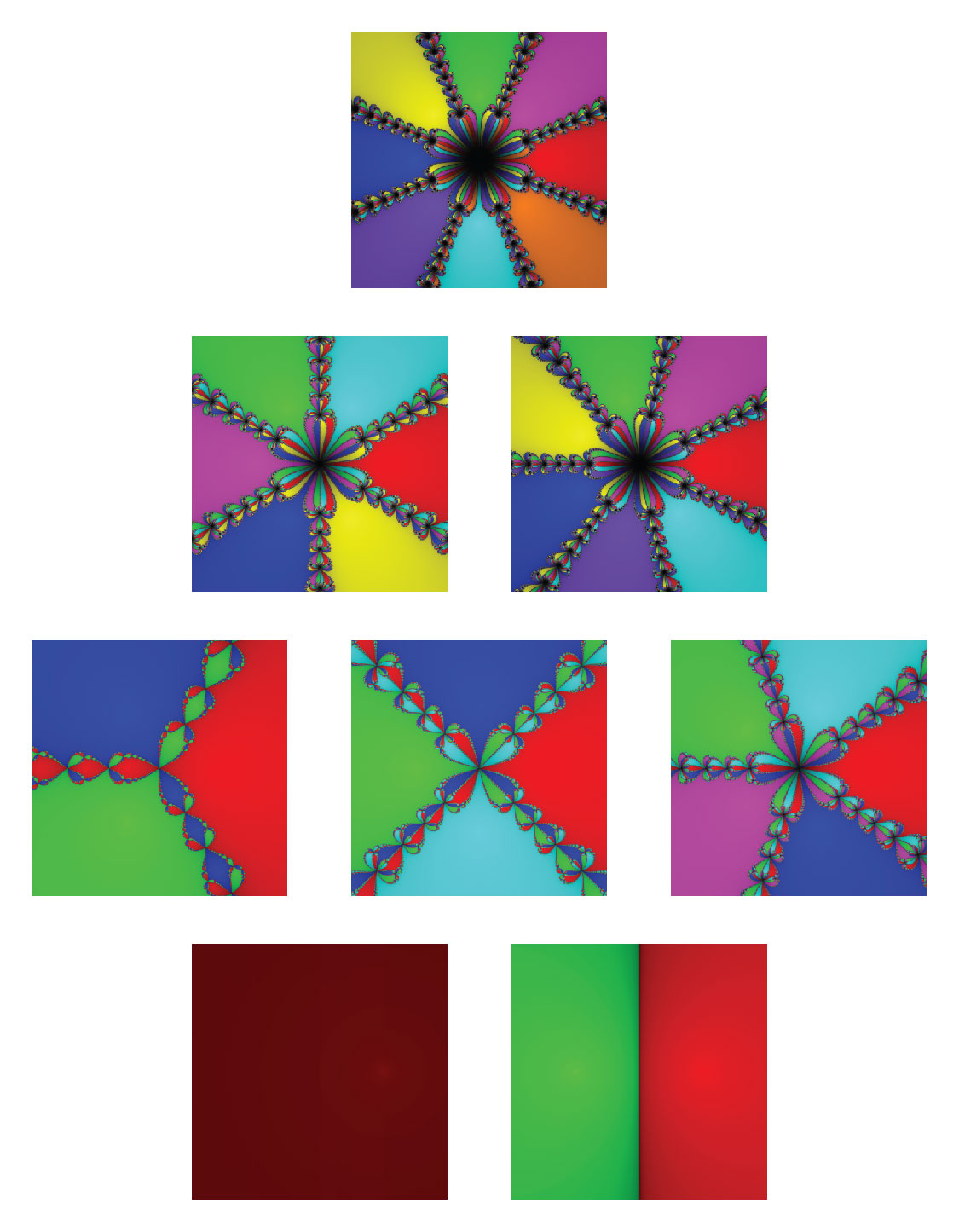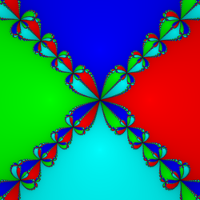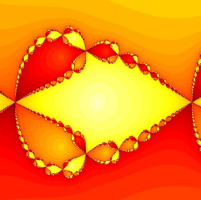# Quarantine Discoveries

### Description and arrangement by Lilly Cordier, Hannah Cui, Salvador Garcia, Morgan Presson, and Hunter Rowe Code by Cruz GodarHow productive were you during quarantine? I bet Issac Newton had you beat when he was in quarantine. Newton discovered many aspects of calculus during the Plague pandemic in the late 1600s while social distancing. Also during this time, he discovered a method for approximating zeros of functions. While the history of zeros of functions dates back thousands of years, all the way back to the time of the ancient Greeks, Newton's method of approximating zeros and its applications were discovered fairly recently only at the end of the 17th century. Prior to Newton’s work, a commonly accepted method of finding zeros resulted in a linear convergence pattern for each iteration. Following the discovery of this method, Newton decided to further study this topic, which led him to discover a new method in which all decimals were kept for each iteration of the zeros of a function, in contrast to previous methods where few decimals were kept. Using this method, the iterations converge quadratically rather than linearly, which drastically improves the accuracy of each iteration. This method is now referred to as Newton’s Method.

It is interesting to note that during the 1665 Plague Outbreak, Newton was a college student and forced to return home. The time he spent at home in quarantine is referred to as his “year of wonders”. Newton actually did some of his most amazing work on differential and integral calculus, the theory of universal gravitation while social distancing (McDonald, 2020). Coincidentally, this project regarding Newton’s Method and Newton fractals was also completed under quarantine due to the coronavirus.

This project further explores Newton’s process of finding approximations of roots for polynomials of different degrees. There is no formula for finding the exact roots of polynomials above a quartic function using only the arithmetic operators: addition, subtraction, multiplication, and division (and by using roots).

### Describing the Math

This topic can easily relate to areas such as mathematical optimization, which is the concept of selecting the best element from a set of available elements. These two ideas are related because Newton’s method allows users to get the closest value to a root which can be considered the ‘best’ or ‘most efficient’ element, which is the same as using optimization to choose the best element from a set that would result in the most ‘efficient’ or ‘effective’ outcome. Newton’s iterations can also relate to numerical analysis and linear algebra, where iterative methods are used in solving systems of equations using matrices. “A numerical method called Gaussian elimination and its variants is simply a precisely stated algorithmic variant of the method of elimination of variables”(Atkinson, 2017)). This leads to an exact solution in a finite number of steps.

Methods like Newton’s iterations and Gaussian elimination are approximation methods that lead to increased accuracy in solutions. Finally, this method is also related to calculus. In our view, the iteration method used in Newton’s method could be thought of as analogous to the integration of a function that serves as a better approximation for the area under a curve. While Riemann sums can provide an approximation of the area under a curve, the limit of these sums provides a far better approximation of area (by integration).

Newton’s method of iterative approximation is useful for finding roots of polynomials. By choosing an initial approximation value, x_0,as a zero of a polynomial f(x), we can find a better approximation with x_1=x_0-(f(x_0))/(f'(x_0)), where f'(x_0)is the derivative of f(x_0). If x_n is an approximation of a solution to f(x)=0and if f'(x)≠0(the derivative of f(x)), then the next approximation is given by x_(n+1)=x_n-(f(x_n))/(f'(x_n)). Repeating recursively, we can find a sequence of values x_n=(x_1,x_2,...x_n) where each iteration results in a better approximation of the root of the polynomial. This process is called the iterative method. Generally, the sequence x_n converges to a root of the polynomial f(Cruzgodar, 2020).

Interestingly, Newton's Method can also be applied to find zeros of complex functions. By fixing a polynomial p(z), Newton’s method can iteratively find the roots of a complex function. Visual representations of this method in the complex plane display patterns called Newton Fractals. Graphing the family of functions defined by p(z) = zn-1 displays particularly interesting patterns because the roots of this polynomial spread evenly around the center and the resulting image is symmetrical. Each color corresponds to a root of the polynomial to which we converge from a given starting point.For example, this image represents the graph of p(z)=z4-1, where the blue, red, teal and green each represent a root of z4-1. Like colors are points that converge to the same root. (Cruzgodar, 2020) Here is a close-up image of a fractal. As we can see, it is the same fractal pattern repeated in smaller and smaller scales forever. This image displays how “when we choose our initial guess for the approximation of a root of a function with Newton’s method, there are initial guesses lying close to each other that converge to similar patterns on different scales” (Krassnigg, 2018-20). In other words, when we look at an image of a Newton fractal, we can zoom in on the image and at some point, we may zoom in enough such that it looks like the original image again.

Our array of images depicts how the roots and powers of polynomials can be applied to visual settings so that we can see and physically comprehend how the number of roots affects the division of space in the complex plane. They show which zero/root every point in the graph converges to by color, and the contours within each colored section represent the number of iterations performed. The accuracy of the iteration increases as the colored section approaches the center, with the very center representing the exact zero. We wanted to explore this topic because changes in the degree result in changes in the boundaries of the roots. Prior to seeing the degree three graph, one may have expected there to be straight-line boundaries between the roots with no fractal designs. However, the graphs of polynomials of degree three and above have fractals at the boundaries of each root. Another aspect of Newton’s method shows that there are some points that do not converge to any root. This can be seen when examining the degree two graph; as there is a grey line between the two roots. As a brief aside, there are points that do not converge in the graphs greater than degree two, but it is very easy to see the non-convergence in degree two. Points that lie along this line do not converge to either root. We learned that this happens as a result of Newton’s method. Examining the polynomial x2-25 and setting x0= 0. By Newton’s method we find (-25)/(2(0)). This computation of division by 0 is not mathematically possible. Therefore, at the boundary of the degree two roots, we see a line of points that do not converge to either root.

There is a rich history behind finding the roots of polynomials. One of the most fundamental mathematical concepts lies in the quadratic formula, which provides a general formula for finding the exact roots of a second-degree polynomial. Formulas that find the exact roots of cubic and quartic functions similar to the quadratic equation can also be found, although no formula using only arithmetic operators and nth roots to find the exact roots of quintic polynomials and polynomials with degrees greater than five exists. In fact, in 1824, Niels Abel proved that it there is no formula for the exact roots of a general degree 5 polynomial using only these operations.

Newton’s method of approximating roots, which works for any degree polynomial, was first published in 1685. In this paper it was stated that “by this means [Newton] shews the number of Roots (real or; imaginary) in every Equation, and the Ingredients of all the Coefficients, in each degree of Affection.” (A Treatise, 1100). This explains how his method effectively approximates the roots of any polynomial, regardless of the degree.

### Related Courses at the University of Oregon

To learn more about the math related to our images, students should learn calculus since the basis of Newton’s method requires an understanding of derivatives. This method can easily be learned and applied in calculus classes, as these classes often utilize methods of finding roots of functions. This topic can also be applied in some computer science classes, as finding roots through Newton’s method can be used to get better approximations of computational values that are often found through the coding of programs.

To learn more about the topics related to Newton’s Method, students could take Math 251, Math 341/342, Math 411/412, and Math 457/557. Math 251 is the first class in the calculus sequence, where students learn how to take derivatives (a key element of Newton’s method). Math 341 and 342 are the classes within linear algebra sequence, in which the complex plane, complex eigenvalues, complex eigenvectors, and an introduction to discrete dynamical systems are discussed. Math 457/557 are called Discrete Dynamical Systems and Newton’s method is included in the course descriptions. Finding the roots of polynomials is an important topic in mathematics, and Newton’s method gives mathematicians a useful tool in understanding these approximations.

### Works Cited

“A Treatise of Algebra, Both Historical and Practical.” Philosophical Transactions of the Royal Society of London, vol. 15, no. 173, 1685, pp. 1095–1106

Atkinson, Kendall E. “Historical Background.” Encyclopædia Britannica, Encyclopædia

Britannica, Inc., 31 May 2017

Burton, A. (2020). Newton's Method and Fractals [PDF]. Walla Walla: Whitman College.

Deuflhard, P. (2012). A Short History of Newton's Method. Documenta Mathematica, 25-30. Retrieved May 21, 2020, from https://www.math.uni-bielefeld.de/documenta/vol-ismp/13_deuflhard-peter.pdf

Godar, Cruz. “Newton's Method.” Cruz Godar, Accessed 16 May 2020,

cruzgodar.com/index.html?page=/applets/newtons-method/newtons-method.html.

Krassnigg, Andreas. “Newton Fractals Explained: Examples and Python Code.”

ComputingSkillSet, 30 Apr. 2020

McDonald, Kerry. “How Isaac Newton Turned Isolation From the Great Plague Into a ‘Year of

Wonders’: Kerry McDonald.” FEE Freeman Article, Foundation for Economic

Education, 27 Mar. 2020

These students’ description has been lightly edited for clarity.